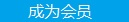您可以捐助，支持我们的公益事业。 1元 10元 50元 认证码：必填求知 文章 文库 Lib 视频 iProcess 课程 认证 咨询 工具 讲座 Modeler Code要资料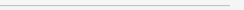订阅捐助
Python: 通过scikit-learn了解机器学习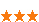892 次浏览     评价： 好 中 差
2018-11-12

 编辑推荐: 本文来自于csdn，文章详细介绍了Supervised Learning(有监督学习)以及ROC曲线等相关知识。

Supervised Learning(有监督学习)

Machine Learning分为有监督学习与无监督学习，这个系列重在介绍有监督学习，即，通过告知算法有关Features和对应的输出Labels，然后当有新的feature数据时，做label预测。

Iris是sklearn中内嵌的一组数据，可以用以学习通过特征值对鸢尾花进行分类。1

 from sklearn.datasets import load_iris iris = load_iris() X = iris.data # numpy.ndarray, Features的那些observation y = iris.target # numpy.ndarray与data的一个label的一一对应

K-nearest neighbors (KNN) classification

 from sklearn.neighbors import KNeighborsClassifier knn = KNeighborsClassifier(n_neighbors=1) knn.fit(X, y) X_new = [[3, 5, 4, 2], [5, 4, 3, 2]] knn.predict(X_new)

 from sklearn.model_selection import train_test_split # 注意，这个模块从0.20开始不存在于 sklearn.cross_validation了! # STEP 1: split X and y into training and testing sets X_train, X_test, y_train, y_test = train_test_split(X, y, test_size=0.4, random_state=4) from sklearn.linear_model import LogisticRegression # STEP 2: train the model on the training set logreg = LogisticRegression() logreg.fit(X_train, y_train) # STEP 3: make predictions on the testing set y_pred = logreg.predict(X_test) # compare actual response values (y_test) with predicted response values (y_pred) from sklearn import metrics print(metrics.accuracy_score(y_test, y_pred))

 # try K=1 through K=25 and record testing accuracy k_range = list(range(1, 26)) scores = [] for k in k_range: knn = KNeighborsClassifier(n_neighbors=k) knn.fit(X_train, y_train) y_pred = knn.predict(X_test) scores.append(metrics.accuracy_score(y_test, y_pred))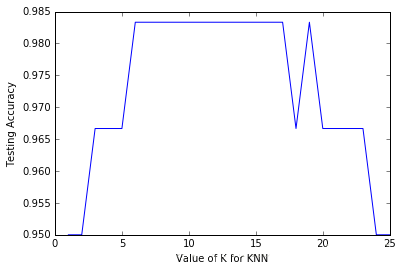Feature选择

K-fold cross-validation

K-fold cross-validation将原始数据均匀地分为K份，每一次将其中一份拿出来做测试数据4，其他参与模型训练，得到一个测试下的匹配值。如此做K次，则有K个测试匹配值，它们的平均值就是平均测试匹配值，平均值最小的模型所使用的参数，即是最佳参数。

 from sklearn.model_selection import cross_val_score # 10-fold cross-validation with K=5 for KNN (the n_neighbors parameter) knn = KNeighborsClassifier(n_neighbors=5) scores = cross_val_score(knn, X, y, cv=10, scoring='accuracy')

 # search for an optimal value of K for KNN k_range = list(range(1, 31)) k_scores = [] for k in k_range: knn = KNeighborsClassifier(n_neighbors=k) scores = cross_val_score(knn, X, y, cv=10, scoring='accuracy') k_scores.append(scores.mean())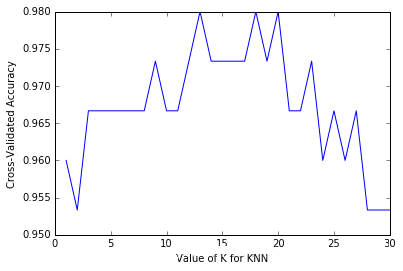from sklearn.grid_search import GridSearchCV # define the parameter values that should be searched k_range = list(range(1, 31)) param_grid = dict(n_neighbors=k_range) # instantiate the grid grid = GridSearchCV(knn, param_grid, cv=10, scoring='accuracy') # fit the grid with data grid.fit(X, y) # examine the best model print(grid.best_score_) # 0.98 print(grid.best_params_) # {'n_neighbors': 13} print(grid.best_estimator_) # KNeighborsClassifier(algorithm='auto', leaf_size=30, metric='minkowski', metric_params=None, n_jobs=1, n_neighbors=13, p=2, weights='uniform')

 k_range = list(range(1, 31)) # 调整参数1 weight_options = ['uniform', 'distance'] # 调整参数2 param_grid = dict(n_neighbors=k_range, weights=weight_options) grid = GridSearchCV(knn, param_grid, cv=10, scoring='accuracy')

GridSearchCV会遍历所有的参数的所有组合，所以非常耗时。要减少遍历数，还有一种做法是采用 RandomizedSearchCV，它只会对组合的一个子集做验证。比如，用户可以通过n_iter=10限制只遍历10个子集。

ROC曲线

ROC曲线，就是在x轴标记，如果实际分类为是的情形下，模型将其错误地标示为是的概率（False Positive Rate）。而y轴则为，如果实际分类是是的情形下，模型也标示是是的概率（True Positive Rate）。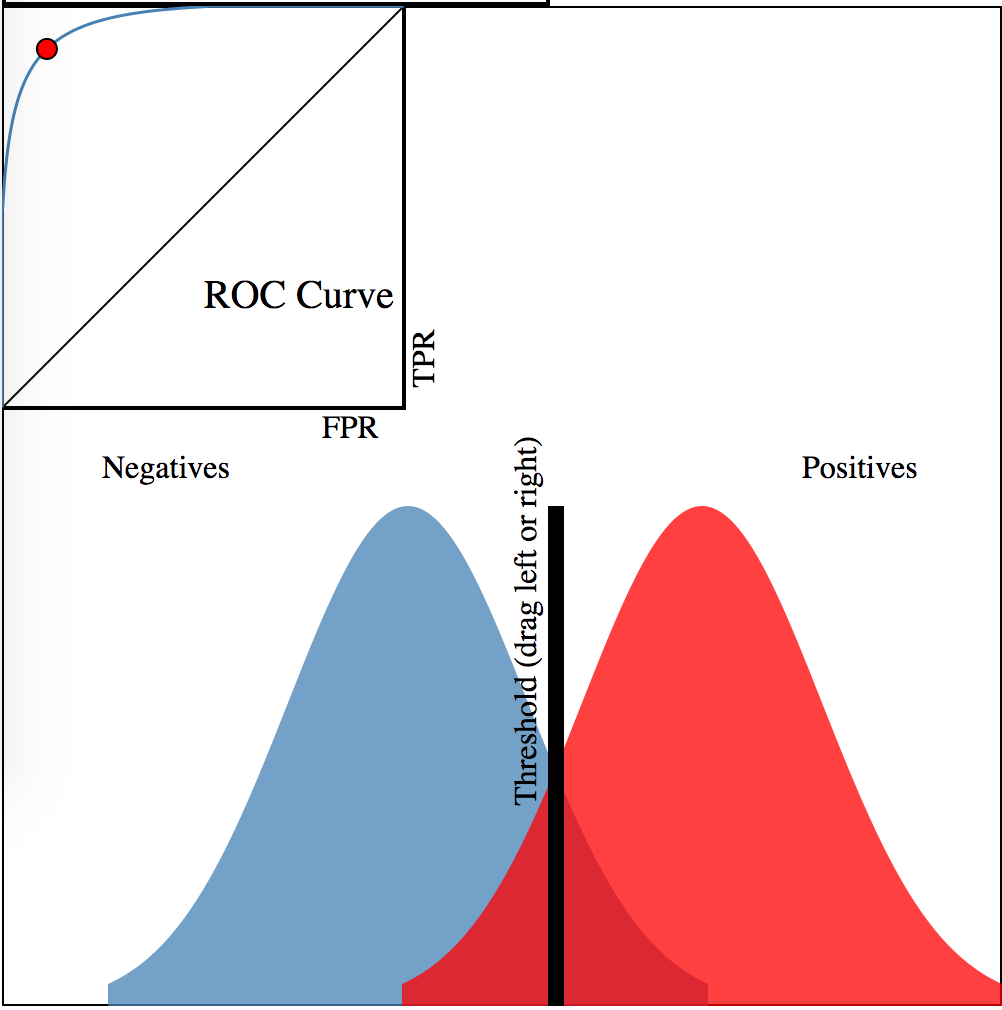X_train, X_test, y_train, y_test = train_test_split(X, y, random_state=0) logreg = LogisticRegression() logreg.fit(X_train, y_train) y_pred_class = logreg.predict(X_test) # IMPORTANT: first argument is true values, second argument is predicted values confusion = metrics.confusion_matrix(y_test, y_pred_class) TP = confusion[1, 1] TN = confusion[0, 0] FP = confusion[0, 1] FN = confusion[1, 0] print(metrics.recall_score(y_test, y_pred_class)) # TPR:(TP / float(TP + FN)) print(FP / float(TN + FP)) # FPR y_pred_prob = logreg.predict_proba(X_test)[:, 1] import matplotlib.pyplot as plt fpr, tpr, thresholds = metrics.roc_curve(y_test, y_pred_prob) plt.plot(fpr, tpr) plt.xlim([0.0, 1.0]) plt.ylim([0.0, 1.0]) plt.title('ROC curve for diabetes classifier') plt.xlabel('False Positive Rate (1 - Specificity)') plt.ylabel('True Positive Rate (Sensitivity)') plt.grid(True) plt.show()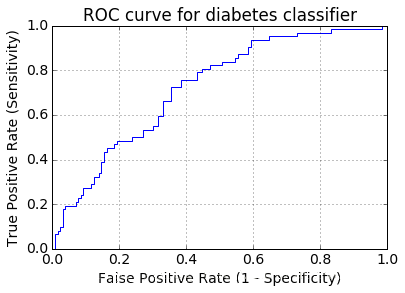# IMPORTANT: first argument is true values, second argument is predicted probabilities print(metrics.roc_auc_score(y_test, y_pred_prob))

 cross_val_score(logreg, X, y, cv=10, scoring='roc_auc').mean()

sklearn有个专门的KFold类，可以通过 from sklearn.cross_validation import KFold 引用。892 次浏览  评价: 好 中 差订阅捐助
 相关文章 我们该如何设计数据库 数据库设计经验谈 数据库设计过程 数据库编程总结
 相关文档 数据库性能调优技巧 数据库性能调整 数据库性能优化讲座 数据库系统性能调优系列
 相关课程 高性能数据库设计与优化 高级数据库架构师 数据仓库和数据挖掘技术 Hadoop原理、部署与性能调优每天2个文档/视频 扫描微信二维码订阅
 订阅技术月刊 获得每月300个技术资源